Courses

# Current Electricity (Part - 1) - Physics, Solution by DC Pandey NEET Notes | EduRev

## JEE : Current Electricity (Part - 1) - Physics, Solution by DC Pandey NEET Notes | EduRev

The document Current Electricity (Part - 1) - Physics, Solution by DC Pandey NEET Notes | EduRev is a part of the JEE Course DC Pandey Solutions for JEE Physics.
All you need of JEE at this link: JEE

INTRODUCTORY EXERCISE 20.1
Ques 1: In the Bohr model of hydrogen atom, the electron is pictured to rotate in a circular orbit of radius 5 × 10-11 m, at a speed 2.2 × 106 m/s. What is the current associated with electron motion?
Ans: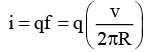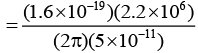= 1.12 × 10-3A = 1.12mA

Ques 2: Copper has one conduction electron per atom. Its density is 8.89 g/cm3 and its atomic mass is 63.54 g/mol. If a copper wire of diameter 1.0 mm carries a current of 2.0 A. What is the drift speed of the electrons in the wire?
Ans:
ρ = 8.89 × 103 kg/m3
Mass of 1 m3 = 8.89 × 103 kg= 8.89 × 103 kg
∴ Number of gram moles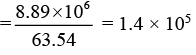Number of atoms = 1.4 × 105 × 6.02 × 1023
= 8.42 × 1028
One atom emits one conduction electron. Therefore number of free electrons in unit volume (or lm3 volume)
n = 8.42 × 1028 per m3
Now i = neA vd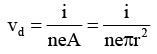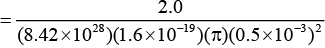= 1.9 × 10-4m/s

Ques 3: When a steady current passes through a cylindrical conductor, is there an electric field inside the conductor?
Ans: Under electrostatic conditions (when no current flows), E = 0. When current is non-zero, then electric field is also non-zero.

Ques 4: Electrons in a conductor have no motion in the absence of a potential difference across it. Is this statement true or false?
Ans: False, there is random or thermal motion of free electrons in the absence of potential difference.

Ques 5: In an electrolyte, the positive ions move from left to right and the negative ions from right to left. Is there a net current? If yes, in what direction?
Ans:
Current due to both is from left to right. So, the two current are additive.

Ques 6: The current through a wire depends on time as, i = (10 + 4t)
Here, i is in ampere and t in seconds. Find the charge crossed through a section in time interval between t = 0 to t = 10 s.

Ans: The resistance of a copper wire and an iron wire at 20°C are 4.1 Ω. and 3.9 Ω respectively. Neglecting any thermal expansion, find the temperature at which resistances of both are equal.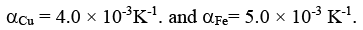INTRODUCTORY EXERCISE 20.2
Ques 1: In household wiring, copper wire 2.05 mm in diameter is often used. Find the resistance of a 35.0 m long wire. Specific resistance of copper is 1.72 ×10-8 Ω-m.
Ans: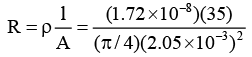= 0.18 Ω

Ques 2: An aluminium wire carrying a current has diameter 0.84 mm. The electric field in the wire is 0.49 V/m. What is,
(a) the current carried by the wire?
(b) the potential difference between two points in the wire 12.0 m apart?
(c) the resistance of a 12.0 m length of this wire? Specific resistance of aluminium is 2.75 × 10-8 Ω-m.
Ans:
(a) In 1 m, potentials difference,
V = 0.49
V = iR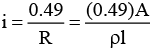= 9.9 A
(b) PD between two points, 12 m apart = (0.49 V/m) (12m) = 5.88 V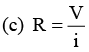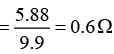Ques 3: A conductor of length l has a non-uniform cross-sect ion. The radius of cross-sect ion varies linearly from a to b. The resistivity of the material is ρ. Find the resistance of the conductor across its ends.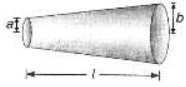Ans:
Radius at distance x from end P,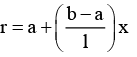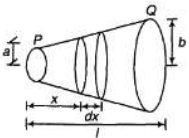Resistance of element of thickness dx is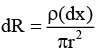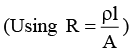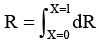Ques 4: The product of resistivity and conductivity of a conductor is constant. Is this statement true or false?
Ans: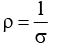ρσ = constant

Ques 5: The resistance of a copper wire and an iron wire at 20°C are 4.1 Ω. and 3.9 Ω respectively. Neglecting any thermal expansion, find the temperature at which resistances of both are equal.
αCu = 4.0 × 10-3K-1. and αFe= 5.0 × 10-3 K-1.
Ans:
4.1[1 + 4.0 × 10-3(θ - 20)]
= 3.9[1 + 5.0 × 10-3(θ - 20)]
Solving we get,
θ = 85°C

INTRODUCTORY EXERCISE 20.3
Ques 1: Find the current through 2Ω and 4Ω resistance.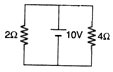Ans:
PD across each resistance is 10V.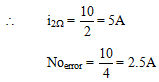Ques 2: In the circuit shown in figure, find the potentials of A, B, C and D and the current through 1Ω and 2Ω resistance.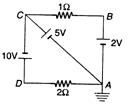Ans:
VA = 0V (as it is earthed)
V- VA = 5W
KC = 5V V- VA = 2V
VB = 2V V- VC = 10V
V= 10 + VC = 15 V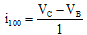= 3A from C to B as K> K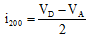= 7.5A from D to A as VD > VA

Ques 3: For what value of E the potential of A is equal to the potential of B?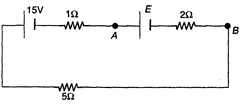Ans:
FA = FB
VAB = 0 or E - ir = 0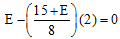Solving this equation we get E = 5V

Ques 4: Ten cells each of emf 1V and internal resistance 1Ω. are connected in series. In this arrangement polarity of two cells is reversed and the system is connected to an external resistance of 2Ω . Find the current in the circuit.
Ans:
Net emf = (n - 2m)E
= (10 - 2 × 2) (1) = 6 V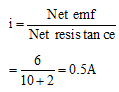Ques 5: In the circuit shown in figure, R1 = R2 = R3 = 10Ω. Find the currents through R1 and R2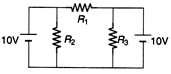Ans: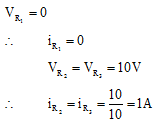Ques 6: Draw:
(b) current versus potential difference graph for a cell.
Ans: (a)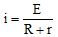i versus R graph is shown in answer.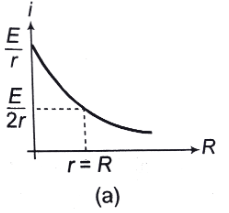(b) V =E - ir
V versus i graph is shown in answer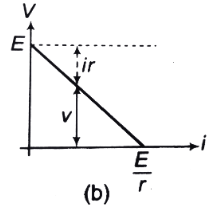Offer running on EduRev: Apply code STAYHOME200 to get INR 200 off on our premium plan EduRev Infinity!

210 docs

,

,

,

,

,

,

,

,

,

,

,

,

,

,

,

,

,

,

,

,

,

,

,

,

;